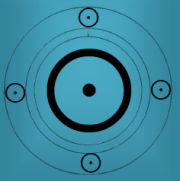Le Pen, Marine

5 August 1968 at 11:20 (= 11:20 AM ) Neuilly sur Seine, France, 48n53, 2e16

Progressed Lunar Phase

4 Oct 2016 23:14:03 GMT New MoonSchauen wir,ob Kronos mich wieder irrt. AC ist Jungfrau, Herrscher Venus   Neumond = pVE = rVE = rPL/rKR pKR = rVE/rKR pJU = rAC/rUR = rSO/rSA = rAC/rPL pUR = rUR pKN = rSO/rKR = rJU pMA = pPL = rME (MC Herrscher) = rMC = rKN/RJU pME (MC Herrscher) = rVE/rKN = rME/rKR pKR = rVE/rKR = rMC/rJU

The new President of the French Republic will be proclaimed on 11 May and undergo their investiture ceremony on 14 May at the latest.

Secondary Progression for 11 May 2017pJU = rMO/rKR pMA = rMO/rME = rAC/rKR pMO = rSO = rVE pSO = pKN = rAC/rJU = rSO/rKN = rME/rJU rME = rPL/rKR = Neumond ( 4.10.2016 ) pPL = rMC = rME (MC Herrscher) = rJU/rKN pVE (AC Herrscher) = rSA = rSO/rUR = rKN/rKR   zwei negative Planetenbilder : pNE = rVE/rUR pSA = rVE/rSA = rVE/rNE   14.Mai kommt dann pMond auf rMA/rSA

Tertiary Progression for 11 May 2017pMO = pMA = pUR = rKR = rMO/rVE pMC = rMC/rKR pVE = pKR = rJU/rNE pPL = pME = pSA = rVE/rKN pJU = rSO/rMA = rPL/rMC

Tertiary Progression for 14 May 2017pSO = rMC = rAc = rMA/rKN = rME/rJU = rWI/rKR = rSO/rKR = pKN/pKR = pVE/pPL = pMA/pUR     Sie wird gewinnen.

02.05.2017